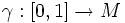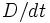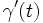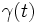# Geodesic for a linear connection

## Definition

### Given data

• A connected differential manifold$M$ with tangent bundle denoted by$TM$.
• A linear connection$\nabla$ for$M$.

### Definition part

Consider a smooth curve$\gamma:[0,1] \to M$. Let$D/dt$ denote the connection along$\gamma$ induced by$\nabla$, and consider the transport along$\gamma$ for the connection$D/dt$. Then, we say that$\gamma$ is a geodesic for$\nabla$ if, under that transport, the tangent vector$\gamma'(0)$ at$\gamma(0)$ gets transported, at time$t$, to the tangent vector$\gamma'(t)$ at$\gamma(t)$.

Equivalently, we say that$\gamma$ is a geodesic if:

$D}{dt}(\gamma'(t)$

(with the derivative interpreted as a suitable one-sided derivative at the endpoints).